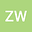Torques and angular momenta of fluid elements in the octonion spaces
•## Abstract

The paper focuses on applying the octonions to explore the influence of the external torque on the angular momentum of fluid elements, revealing the interconnection of the external torque and the vortices of vortex streets. J. C. Maxwell was the first to introduce the quaternions to study the physical properties of electromagnetic fields. The contemporary scholars utilize the quaternions and octonions to investigate the electromagnetic theory, gravitational theory, quantum mechanics, special relativity, general relativity and curved spaces and so forth. The paper adopts the octonions to describe the electromagnetic and gravitational theories, including the octonionic field potential, field strength, linear momentum, angular momentum, torque and force and so on. In case the octonion force is equal to zero, it is able to deduce eight independent equations, including the fluid continuity equation, current continuity equation, and force equilibrium equation and so forth. Especially, one of the eight independent equations will uncover the interrelation of the external torque and angular momentums of fluid elements. One of its inferences is that the direction, magnitude and frequency of the external torque must impact the direction and curl of the angular momentum of fluid elements, altering the frequencies of Karman vortex streets within the fluids. It means that the external torque is interrelated with the velocity circulation, by means of the liquid viscosity. The external torque is able to exert an influence on the direction of downwash flows, improving the lift and drag characteristics generated by the fluids.
10 Aug 2021Submitted to Mathematical Methods in the Applied Sciences
11 Aug 2021Submission Checks Completed
11 Aug 2021Assigned to Editor
25 Aug 2021Reviewer(s) Assigned
10 Dec 2021Review(s) Completed, Editorial Evaluation Pending
11 Dec 2021Editorial Decision: Revise Major